# Floor function

(diff) ← Older revision | Latest revision (diff) | Newer revision → (diff)

entier function, greatest integer function, integral part function

The function of a real variable that assigns to a real numberthe largest integer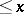. The modern notation is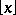; the classical notation is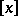. In computer science and computer languages it is often denoted by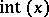.

The related ceiling function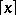gives the smallest integer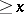. The fractional part function is defined as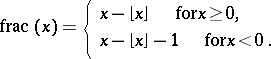The nearest integer function is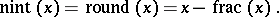How to Cite This Entry:
Floor function. Encyclopedia of Mathematics. URL: http://encyclopediaofmath.org/index.php?title=Floor_function&oldid=33154
This article was adapted from an original article by M. Hazewinkel (originator), which appeared in Encyclopedia of Mathematics - ISBN 1402006098. See original article